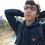# Integral Proofs for Consideration: GammaI saw these definite integrals in a standard mathematical table, can someone provide proofs to these so that we can all learn the interesting methods to solving these integrals?

1. $\Large{\int_{0}^{1}{ (\log { \dfrac{1}{x} })^n }\, dx = n! \quad\quad n > -1 }$

2. $\Large{\int_{0}^{\infty}{\dfrac{1}{x}(\dfrac{1}{1+x^k}-e^{-x})}\, dx = \gamma \quad\quad k>0 }$

3. $\Large{\int_{0}^{\frac{\pi}{2}}{\sqrt{\cos{x} } } \, dx = \dfrac{(2\pi)^\frac{3}{2} }{ (\Gamma(\frac{1}{4} ) )^2 } }$

4. $\Large{\int_{0}^{1}{ \ln { \Gamma(q)} }\, dq =\ln{ \sqrt{2\pi}}}$

5. $\Large{\int_{0}^{\infty}{\dfrac{ \operatorname{W}(x)}{x\sqrt{x}} }\, dx =2\sqrt{2\pi}}$

6. $\large{\int_{-\infty}^{\infty}\frac{e^{i nx}}{\Gamma(\alpha+x) \Gamma(\beta -x)}dx = \frac{ \left(2\cos \frac{n}{2} \right)^{\alpha +\beta-2}}{\Gamma(\alpha+\beta-1)}e^{\frac{in}{2}(\beta - \alpha)} \quad |n|<\pi \quad \text{and} \quad \Re(\alpha+\beta)>1}$

For clarity:

$\large{ \Gamma(x)}$ =The Gamma Function

$\large{ \ln { \Gamma(x)}}$=The Log-Gamma Function

$\large{\gamma}$ =The Euler-Mascheroni Constant

$\large{\operatorname{W}(x)}$ = Lambert W-Function

$\large{e^x}$ = Natural Exponential Function

$\large{\pi}$ = Pi

Thank you to everyone who helps!Note by Hussein Hijazi
5 years ago

This discussion board is a place to discuss our Daily Challenges and the math and science related to those challenges. Explanations are more than just a solution — they should explain the steps and thinking strategies that you used to obtain the solution. Comments should further the discussion of math and science.

When posting on Brilliant:

• Use the emojis to react to an explanation, whether you're congratulating a job well done , or just really confused .
• Ask specific questions about the challenge or the steps in somebody's explanation. Well-posed questions can add a lot to the discussion, but posting "I don't understand!" doesn't help anyone.
• Try to contribute something new to the discussion, whether it is an extension, generalization or other idea related to the challenge.

MarkdownAppears as
*italics* or _italics_ italics
**bold** or __bold__ bold
- bulleted- list
• bulleted
• list
1. numbered2. list
1. numbered
2. list
Note: you must add a full line of space before and after lists for them to show up correctly
paragraph 1paragraph 2

paragraph 1

paragraph 2

[example link](https://brilliant.org)example link
> This is a quote
This is a quote
    # I indented these lines
# 4 spaces, and now they show
# up as a code block.

print "hello world"
# I indented these lines
# 4 spaces, and now they show
# up as a code block.

print "hello world"
MathAppears as
Remember to wrap math in $$ ... $$ or $ ... $ to ensure proper formatting.
2 \times 3 $2 \times 3$
2^{34} $2^{34}$
a_{i-1} $a_{i-1}$
\frac{2}{3} $\frac{2}{3}$
\sqrt{2} $\sqrt{2}$
\sum_{i=1}^3 $\sum_{i=1}^3$
\sin \theta $\sin \theta$
\boxed{123} $\boxed{123}$

Sort by:

1. $u=\ln(x^{-1})\to x=e^{-u}\to dx=-e^{-u} du$. the boundaries: $\mid_0^1\to \mid_\infty^0$. we get the integral $\int_\infty^0 u^n(-e^{-u})du=\int_0^\infty u^ne^{-u} du =\Gamma(n+1)=n!$

- 4 years, 4 months ago

bump

- 5 years ago

@Calvin Lin How can I center the links with the functions and constants? It always starts a new paragraph and I believe thats because I closed the LaTeX boundaries, but I did that so I could add a link :S

- 5 years ago

We currently do not allow for linking within Latex.

I've edited the first line accordingly, where it is not centered (and I don't see a strong reason for doing so).

Staff - 5 years ago

Thanks! Got it organized now :)

- 5 years ago